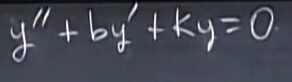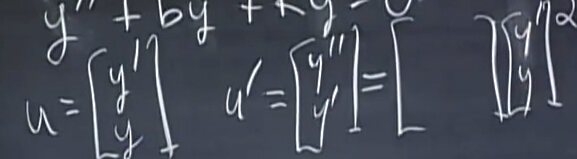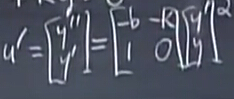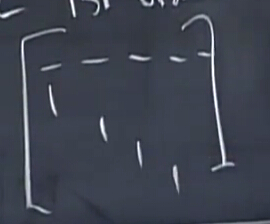# 一阶常系数微分方程

## Au=$Au=$dudt$du\over dt$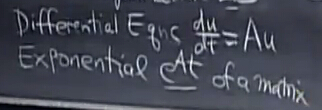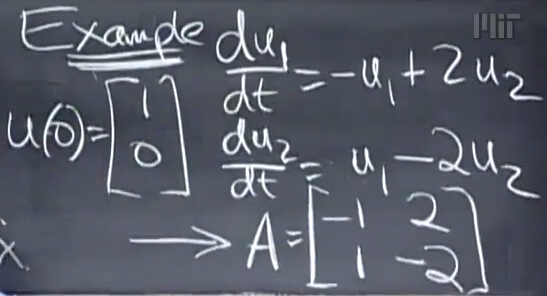### 特征值与特征向量

• 行列式determinant为特征值的积
• 矩阵的迹trace为特征值的和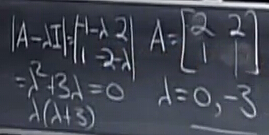• 对于特征值为0，特征向量即为null space，free variable自由变量置1很容易求得
• 对于另一个特征值-3，利用AλI$A-\lambda I$特征向量不变，也可以转换为求解null space

## 解的形式λ1eλ1tx1=Aeλ1tx1$\Rightarrow\lambda_1e^{\lambda_1t}x_1=Ae^{\lambda_1t}x_1$
λ1x1=Ax1$\Rightarrow\lambda_1x_1=Ax_1$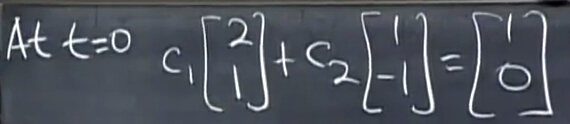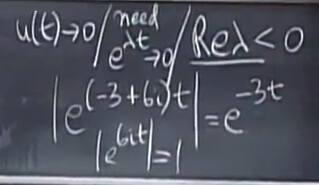## 特征分解

dudt$du\over dt$=Au$=Au$
S$\Rightarrow S$dvdt$dv\over dt$=ASv$=ASv$
$\Rightarrow$dvdt$dv\over dt$=S1ASv$=S^{-1}ASv$
$\Rightarrow$dvdt$dv\over dt$=Λv$=\Lambda v$
$\Rightarrow$dv1dt$dv_1\over dt$=Λv1$=\Lambda v_1$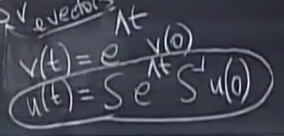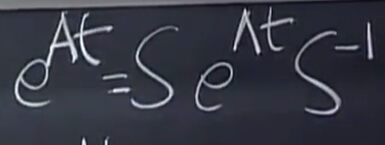## 关于eAt$e^{At}$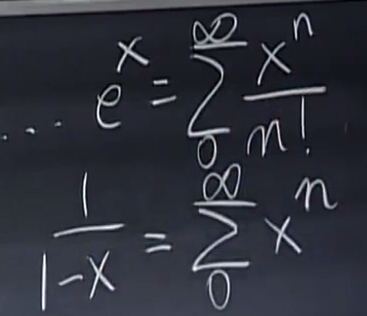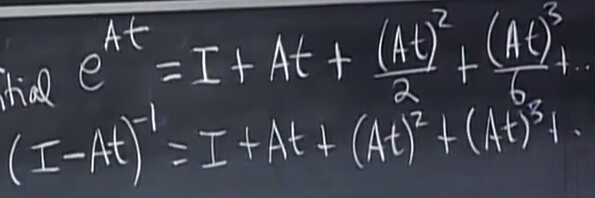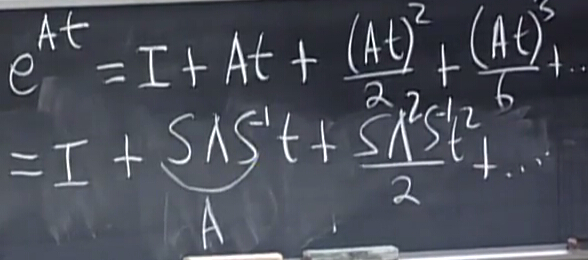I=SS1$I=SS^{-1}$，化简得eAt=SeλtS1$e^{At}=Se^{\lambda t}S^{-1}$，这些公式成立的前提是A$A$可以对角化.

u(t)=SeΛtS1u(0)$u(t)=Se^{\Lambda t}S^{-1}u(0)$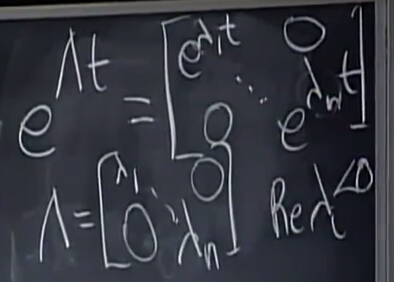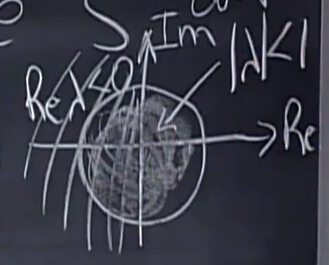# 关于二阶微分方程